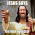## Wednesday, November 5, 2014

### NFL Picks Contest- Week 9 Results and Standings

Here are the Week 9 results and standings in the NFL Picks Contest. OneAndDone stays on top. Captain Crunch and lightning36 inch a little closer.

angerisagift- Minnesota (W 29-26) = 13 pts.
MOJO- Seattle (W 30-24) = 16 pts.
Vegas Vic- Cincinnati (W 33-23) = 20 pts.
Captain Crunch- Kansas City (W 24-10) = 24 pts.
OneAndDone- Seattle (W 30-24) = 16 pts.
mrben09- San Francisco (L 10-13) = (3 pts.)

ggrouchie- Kansas City (W 24-10) = 24 pts.
Coach- Seattle (W 30-24) = 16 pts.
TeamMiRketti-  Cincinnati (W 33-23) = 20 pts.
lightning36- Cincinnati (W 33-23) = 20 pts.
Neophyte- Kansas City (W 24-10) = 24 pts.

Music City Momma- San Francisco (L 10-13) = (3 pts.)
TwoMinuteWarning- Cincinnati (W 33-23) = 20 pts.
Grange95-  Seattle (W 30-24) = 16 pts.
M Prosk- Cincinnati (W 33-23) = 20 pts.
cokeboy99- Seattle (W 30-24) = 16 pts.

Here are the current standings;

OneAndDone- 27+17+17+34+41+5+31+38+16 = 226 pts.
MOJO-  27+17+17+29+16 +24+32+37+16 = 215 pts.
Captain Crunch- 27+17+17+29+16+5+35+38+24 = 208 pts.
lightning36- 27+17+17+29+16+24+31+24+20 = 205 pts.
mrben09- 27+17+17+29+16+24+31+37-3 = 195 pts.
ggrouchie- 27+17+17+34+41+13+20-(3)+24 = 190 pts.

TwoMinuteWarning- (3)+17+37+29+16-(7)+35+37+20 = 181 pts.
cokeboy99- 27-(8)+21+34+16+13+20+38+16 = 177 pts.
Coach- 27+17+21+29+20+24+20-(3)+16 = 171 pts.
Vegas Vic- 27+17+21+29+16+5+32-(3)+20 = 164 pts

Music City Momma- 13+17+21+29+16-(7)+32+38-(3) = 156 pts.
Grange95- (3)+17+21+29+16+24+11+20+16 = 151 pts.
M Prosk- (3)+ 0+ 0+34+41+13+11+20+20 = 136 pts.
Neophyte- 27+17-(19)+0+41+24+21+0+24 = 135 pts.
TeamMiRketti- 27+17+17-(3)+16+24+0-(19)+20 = 99 pts.

Thanks for playing.

Week 10 picks are due by Saturday at 6pm (CDT). Only Sunday and Monday games are eligible.

Good luck!

#### 1 comment:

1.Ravens brother just in case u didnt get email. hope all is weel with yr mom. OPEN CARRY TEXAS Biiiiiiiiiiiiiiiiiiiitches